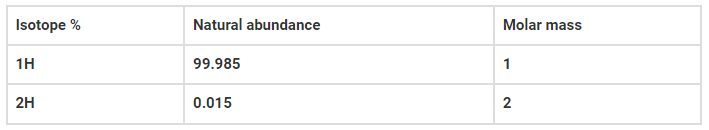# Calculate the average atomic massQuestion:

Calculate the average atomic mass of hydrogen using the following data :Solution:

Average atomic mass = 99.985*1+0.015*2/100

=099.985*1+0.015*2/100

=1.00015u